# Test: Measurement And Error Analysis

## 10 Questions MCQ Test Physics Class 11 | Test: Measurement And Error Analysis

Description
This mock test of Test: Measurement And Error Analysis for Class 11 helps you for every Class 11 entrance exam. This contains 10 Multiple Choice Questions for Class 11 Test: Measurement And Error Analysis (mcq) to study with solutions a complete question bank. The solved questions answers in this Test: Measurement And Error Analysis quiz give you a good mix of easy questions and tough questions. Class 11 students definitely take this Test: Measurement And Error Analysis exercise for a better result in the exam. You can find other Test: Measurement And Error Analysis extra questions, long questions & short questions for Class 11 on EduRev as well by searching above.
QUESTION: 1

### The accuracy in the measurement of time for 20 oscillations of pendulum for the following measurements, t1 = 39.6 s, t2 = 39.9 s and t3 = 39.5 s is

Solution:

Given,
t1 = 39.6 s
t2 = 39.9s
t3 = 39.5 s
Least count of measuring instrument = 0.1 s
[As measurements have only one decimal place] Percision in the measurement  = Least count of the measuring instrument =0.1 s
Mean value of time for 20 oscillations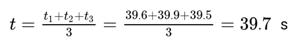Absolute errors in the measurements
Δt1 = t - t1 = 39.7 - 39.6 = 0.1s
Δt2 = t - t2 = 39.7 - 39.9 = -0.2 s
Δt3 = t - t3 = 39.7 - 39.5 = 0.2 s

Mean absolute error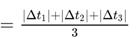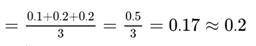∴ Accuracy of measurement =±0.2s.

QUESTION: 2

### Which of the following is caused by careless handling?

Solution:

Gross errors are mostly due to lack of knowledge, judgment and care on the part of experiment. That is Gross error is caused by careless handling.

QUESTION: 3

### A student measures the distance covered and time taken by a body under free fall which was intially at rest. He uses this data for estimating g, the acceleration due to gravity. If the maximum percentage error in measurement  of the distance and the time are e1 and e2 respectively, then the maximum possible percentage error in the estimation of g is

Solution:

From the releation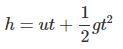h= 1/2gt ⇒ g = 2h/t2

Taking natural logarithms on both sides, we get

ln (g)  = ln (h) - 2ln (t) + ln (2)

Differentiating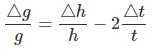for maximum permissible errors , we add both the errors . so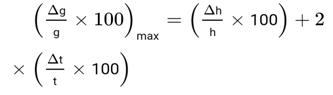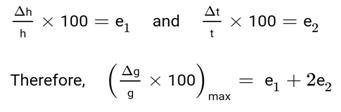QUESTION: 4

Error in the measurement of radius of a sphere is 1%. The error in the calculated value of its volume is

Solution:

V=4πr3/3

% error is volume=3×error in radius   =3×1= 3%

QUESTION: 5

The error in two readings of A are 0.01 and -0.03, then mean absolute error is?

Solution:

absolute error = 0.01-0.03 /2 = -0.01

Mean absolute error = (|0.01 -(-0.01)|+|-0.03-(-0.01)|)/2
= (0.02+0.02)/2

= 0.02

QUESTION: 6

At a certain place, the acceleration due to gravity is determined using a simple pendulum. The length of the pendulum is 25.0 cm and a stopwatch with least count is 1 s measures the time taken by the pendulum for 40 oscilations to be 50 s. the accuracy in the measurement of g is

Solution: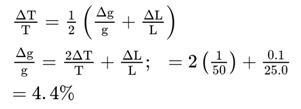QUESTION: 7

The most suitable instrument for measuring the size of an atom is.

Solution:

Only electron microscope has resolution of 0.5-0.6 Angstrom which is less than the size of an atom.

QUESTION: 8

Which is the most accurate atomic clock?

Solution:

Cesium 133 is the element most commonly chosen for atomic clocks.

QUESTION: 9

The method used to measure the distance of a planet from the earth is

Solution:

The astronomers mainly use parallax method to find distances to objects like other planets. To calculate the distance to a star or to a planet, astronomers observe it from different places along Earth's orbit around the Sun.

*Answer can only contain numeric values
QUESTION: 10

What is the percentage error in the physical quantity A if it is related to four other physical quantities a, b, c and d as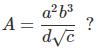The percentage error in measurement of a, b, c and d are 1%, 3%, 2% and 1% respectively.

Solution:

Multiply the exponents with their respective bases and add them. Remember that, error can only be added and not subtracted.

= 2 x a + 3 x b + 1 x d + 1/2 x c

= 2 x 1% + 3 x 3% + 1 x 1% + 1/2 x 2%

= 2% + 9% + 1% + 1%

= 13%